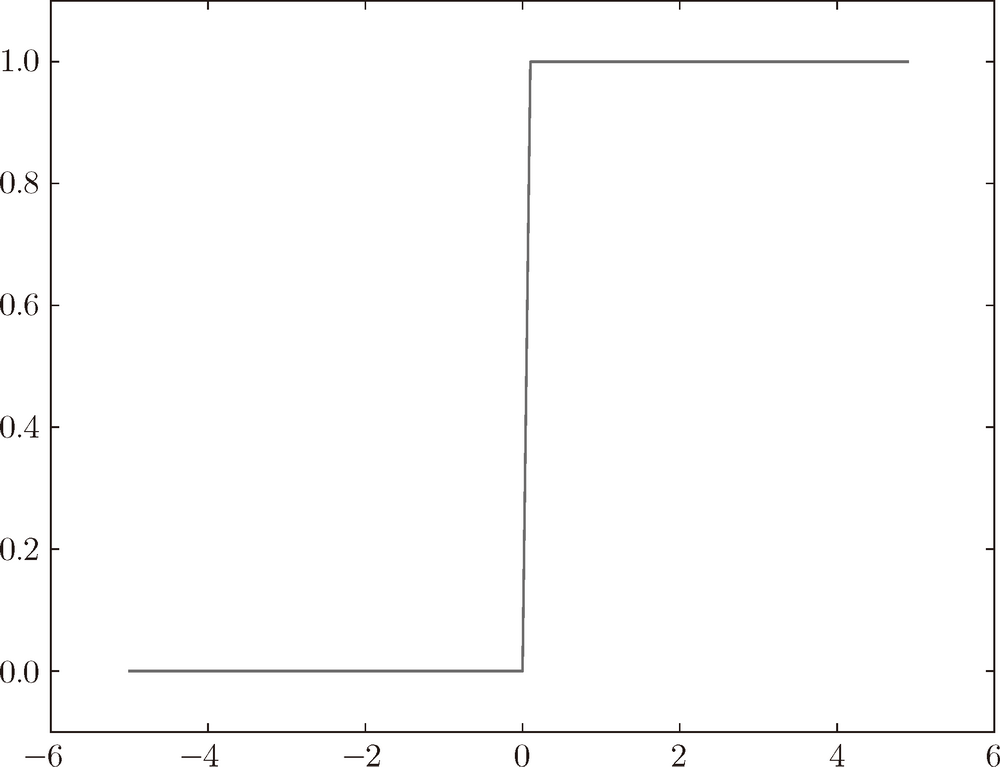# 深度学习入门：基于 Python 的理论与实现 (7)：神经网络 3.2.3(阶跃函数的图形)

import numpy as npimport matplotlib.pylab as plt def step_function(x):    return np.array(x > 0, dtype=np.int) x = np.arange(-5.0, 5.0, 0.1)y = step_function(x)plt.plot(x, y)plt.ylim(-0.1, 1.1) #  指定 y 轴的范围plt.show()

np.arange(-5.0, 5.0, 0.1) 在 -5.0 到 5.0 的范围内，以 0.1 为单位，生成 NumPy 数组（[-5.0, -4.9,…, 4.9]）。step_function() 以该 NumPy 数组为参数，对数组的各个元素执行阶跃函数运算，并以数组形式返回运算结果。对数组 xy 进行绘图，结果如图 3-6 所示。